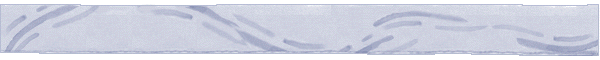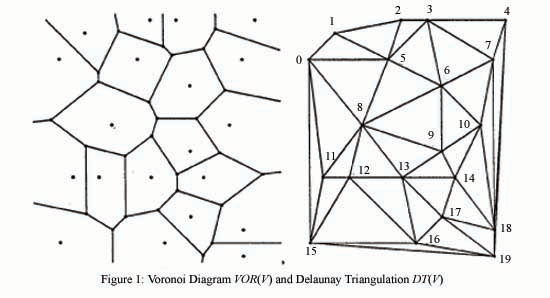Percolation Theory and Forest FiresForest Fire Modeling

Percolation has been used to model fire spread on a square lattice, each square representing a tree earlier as one of the Applications of Percolation Theory. Here we represent the forest as a Voronoi Network.

The fire spread network (graph) F(V,A) with vertex set V and arc set A represents the possibilities of fire spread in a region. The vertices will correspond to the centers of polygon cells in the region, and the directed arcs will represent the possibility of fire spread from one cell to another. A cell represents a homogeneous forest area in terms of several static attributes, such as fuel type, elevation and slope. F(V,A) can be defined with remote sensing and GIS software. See for example the following two figures which first represent the GIS Voronoi cellular polygon network VOR(V) and the figure on the right the fire spread network which is based on the Delaunay triangulation DT(V). Our model of the fire spread network is a Finite Percolation Network based on DT(V).